# MCAT Physical : Solubility and Solution Equilibrium

## Example Questions

← Previous 1

### Example Question #1 : Solubility And Solution Equilibrium

Which of the following compounds would make a weak electrolyte in solution?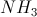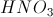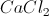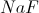Explanation:

A strong electrolyte is a compound that will conduct electricity while in solution. This is because it creates many charged ions in the solution. Because we want a weak electrolyte, the answer will be the compound that does not form many ions when in solution. NHis a weak base which does not dissociate completely. As a result, it creates few ions in solution, making it a weak electrolyte.

The other answer choices represent either ionic compounds or strong acids, and will dissociate completely in solution.

### Example Question #2 : Solubility And Solution Equilibrium

Which of the following compounds makes the least effective electrolyte?

Sodium chloride

Ammonium carbonate

Silver chloride

Magnesium bromide

Silver chloride

Explanation:

An electrolyte is a substance that creates ions when in solution. These compounds conduct electricity very well by donating ions to an aqueous solution. The worst electrolyte will be the compound that creates the smallest amount of ions in solution. Silver chloride is relatively insoluble in solution, meaning that it will make the smallest amount of ions out of the given options.

Van't Hoff factor can also be useful in determining electrolyte effectiveness, but is irrelevant in this particular question.

### Example Question #3 : Solubility And Solution Equilibrium

Which of the following is present in a solution of sodium cyanide?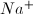ions,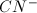ions, and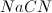moleculesions andmolecules, but noionsmolecules, but almost noorionsandions, but almost nomoleculesions andmolecules, but noionsandions, but almost nomolecules

Explanation:

Salts are a type of strong electrolyte, as are strong acids and strong bases. Strong electrolytes break up completely into ions (or "ionize") when dissolved in water. A solution of sodium cyanide would contain almost entirely sodium and cyanide ions, and no salt molecules.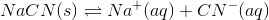### Example Question #4 : Solubility And Solution Equilibrium

Barium fluoride dissolves in solution according to the following equation.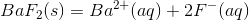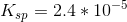Enough BaF2 is added to create a saturated liter of aqueous solution.

Suppose that 0.5M of NaF is added to the solution. What will the solubility of BaF2 be in the new solution?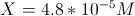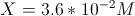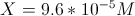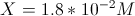Explanation:

When 0.5M of NaF is added, it increases the amount of fluoride ions in the solution. As a result, we can expect the solubility of the salt to decrease (a result of the common ion effect).

To determine by how much the solubility will decrease, we must use the solubility product constant expression with the newfound fluoride concentration. The expression for the equation is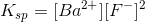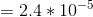. Remeber that pure solids and liquids (such as solid barium flouride are not included in this expression).

Since there is a starting concentration of 0.5M for the fluoride, the predicted increases in the ion concentrations for Ba2+ and F- are now X and (0.5 + X) respectively. We can plug these variables into out equation.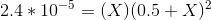Since the value of X will be much smaller than 0.5, it is acceptable to simplify the fluoride portion of the equation to just (0.5)2.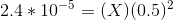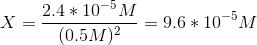### Example Question #5 : Solubility And Solution Equilibrium

Barium fluoride dissolves in solution according to the following equation.Assume an aqueous solution is saturated with BaF2. What is the solubility of BaF2?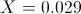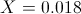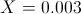It depends on the concentration of BaF2 in the solution.Explanation:

When the solution is saturated, we can determine the solubility of the salt in the solution. We can determine the solubility by using the solubility product constant and comparing it to the amount of ions predicted to be created in the dissolving of the salt. Since the dissolving of BaFwill yield 1 Ba2+ ion and 2 F- ions, we can express their increases as X and 2X respectively. Plugging this in to the equation for the solubility product constant yields the calculations below.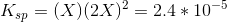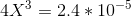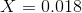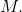### Example Question #6 : Solubility And Solution Equilibrium

Scandium hydroxide,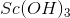, has a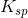value of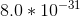. What is its solubility?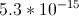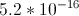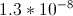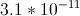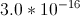Explanation:

Scandium hydroxide dissociates according to the following reaction.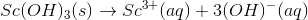Thefor this dissociation is written as follows. Note that the solid is left out of the equation.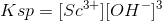Ifis the amount of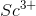that forms whendissociates, thenis the amount of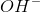formed, based on stoichiometry.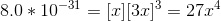Solving this equation gives us the value of.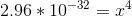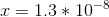This value is the solubility of scandium hydroxide.

### Example Question #11 : Solubility And Ions

Which substance is more soluble in hydrochloric acid than sodium hydroxide?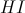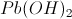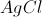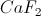Explanation:

Though we are asking about solubility in a strong acid and in a strong base, this is not an acid-base chemistry question.

Instead, we are dealing with solubility, which is directly affected by the solubility product constant. The solubility product constant for each answer choice is given below.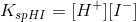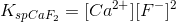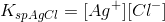Hydrochloric acid will contribute to hydrogen and chloride ion concentrations, while sodium hydroxide will contribute to sodium and hydroxide ion concentrations. The solubility product constant for hydroiodic acid incorporates the hydrogen ions, while the product constant for lead (II) hydroxide incorporates the hydroxide ions.

Increasing the ion concentration results in the common ion effect. Compounds are less soluble in solutions already containing ions that contribute to their solubility product constant. We can thus conclude that lead (II) hydroxide will be less soluble in sodium hydroxide because the sodium hydroxide contributes a common ion.

### Example Question #8 : Solubility And Solution Equilibrium

Electronegativity is an important concept in physical chemistry, and often used to help quantify the dipole moment of polar compounds. Polar compounds are different from those compounds that are purely nonpolar or purely ionic. An example can be seen by contrasting sodium chloride, NaCl, with an organic molecule, R-C-OH. The former is purely ionic, and the latter is polar covalent.

When comparing more than one polar covalent molecule, we use the dipole moment value to help us determine relative strength of polarity. Dipole moment, however, is dependent on the electronegativity of the atoms making up the bond. Electronegativity is a property inherent to the atom in question, whereas dipole moment is a property of the bond between them.

For example, oxygen has an electronegativity of 3.44, and hydrogen of 2.20. In other words, oxygen more strongly attracts electrons when in a bond with hydrogen. This leads to the O-H bond having a dipole moment.

When all the dipole moments of polar bonds in a molecule are summed, the molecular dipole moment results, as per the following equation.

Dipole moment = charge * separation distance

Given the polarity of O-H bonds in water described in the passage, what is the Ksp expression for the following reaction, assuming it takes place in an aqueous environment?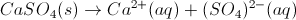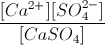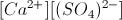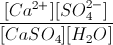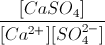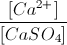Explanation:

The Ksp is a standard equilibrium constant for the dissolution reaction of a solute. As such, it follows the standard formula for equilibrium constants, and excludes pure liquids and solids (like water and calcium sulfate!).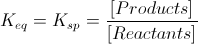### Example Question #9 : Solubility And Solution Equilibrium

Barium fluoride dissolves in solution according to the following equation.Write the solubility product constant (Ksp) for the reaction.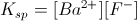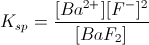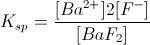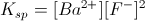Explanation:

When writing the solubility product for a reaction, it is important to remember that solid and liquid compounds are not included in the expression. As a result, barium fluoride would not be included, because it is a solid. Just like the law of mass action, coefficients are the exponents in the expression.

Since only the products are used in the expression (the reactant is a pure solid), it is written as.

### Example Question #10 : Solubility And Solution Equilibrium

Which of the following is true of a saturated solution?

The ionization product is greater than the Ksp

Salt will spontaneously precipitate out of the solution

The solute will continue to dissolve.

The solution is at equilibrium